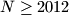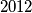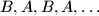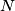### IMO Shortlist 2012 problem C4

Kvaliteta:
Avg: 0,0
Težina:
Avg: 7,0
Players$A$ and$B$ play a game with$N \geq 2012$ coins and$2012$ boxes arranged around a circle. Initially$A$ distributes the coins among the boxes so that there is at least$1$ coin in each box. Then the two of them make moves in the order$B,A,B,A,\ldots$ by the following rules:
(a) On every move of his$B$ passes$1$ coin from every box to an adjacent box.
(b) On every move of hers$A$ chooses several coins that were not involved in$B$'s previous move and are in different boxes. She passes every coin to and adjacent box.
Player$A$'s goal is to ensure at least$1$ coin in each box after every move of hers, regardless of how$B$ plays and how many moves are made. Find the least$N$ that enables her to succeed.
Izvor: Međunarodna matematička olimpijada, shortlist 2012